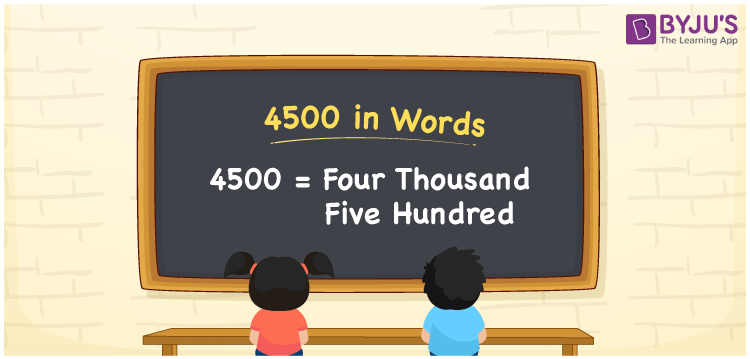# 4500 in Words

In English, the number 4500 is represented as four thousand five hundred. The number name 4500 is written using the ones, tens, and hundreds places of the number. As a result, when writing the number 4500 in words, the place value table helps a lot. For instance, if you paid Rs. 4500 for a garment, you may write “I paid Rs. Four thousand five hundred for a garment.” In this article, we’ll discuss how to spell 4500 in English as well as how to write the number 4500 in words with a complete explanation.

 4500 in Words Four Thousand Five Hundred Four Thousand Five Hundred in Numbers 4500

## 4500 in English Words## How to Write 4500 in Words?

Determine the place value of each digit in the given number while writing 4500 in Words. The following is the place value chart for the number 4500:

 Thousands Hundreds Tens Ones 4 5 0 0

As a result, the expanded form of the number 4500 is:

=4 × Thousand + 5 × Hundred + 0 × Ten + 0 × One

= 4 × 1000 + 5 × 100 + 0 × 10 + 0 × 1

= 4500

= Four thousand five hundred

Hence, 4500 in words is Four thousand five hundred.

4500 is a natural number that is one less than 4501 and one higher than 4499.

4500 in words – Four thousand five hundred

Is 4500 an odd number? – No

Is 4500 an even number? – Yes

Is 4500 a perfect square number? – No

Is 4500 a perfect cube number? – No

Is 4500 a prime number? – No

Is 4500 a composite number? – Yes

## Frequently Asked Questions on 4500 in Words

Q1

### Write 4500 in words.

4500 in words is four thousand five hundred.

Q2

### Simplify 4300+200, and express in words.

Simplifying 4300+200, we get 4500. Therefore, 4500 in words is four thousand five hundred.

Q3

### How to write four thousand five hundred in numbers?

Four thousand five hundred in numbers is 4500.